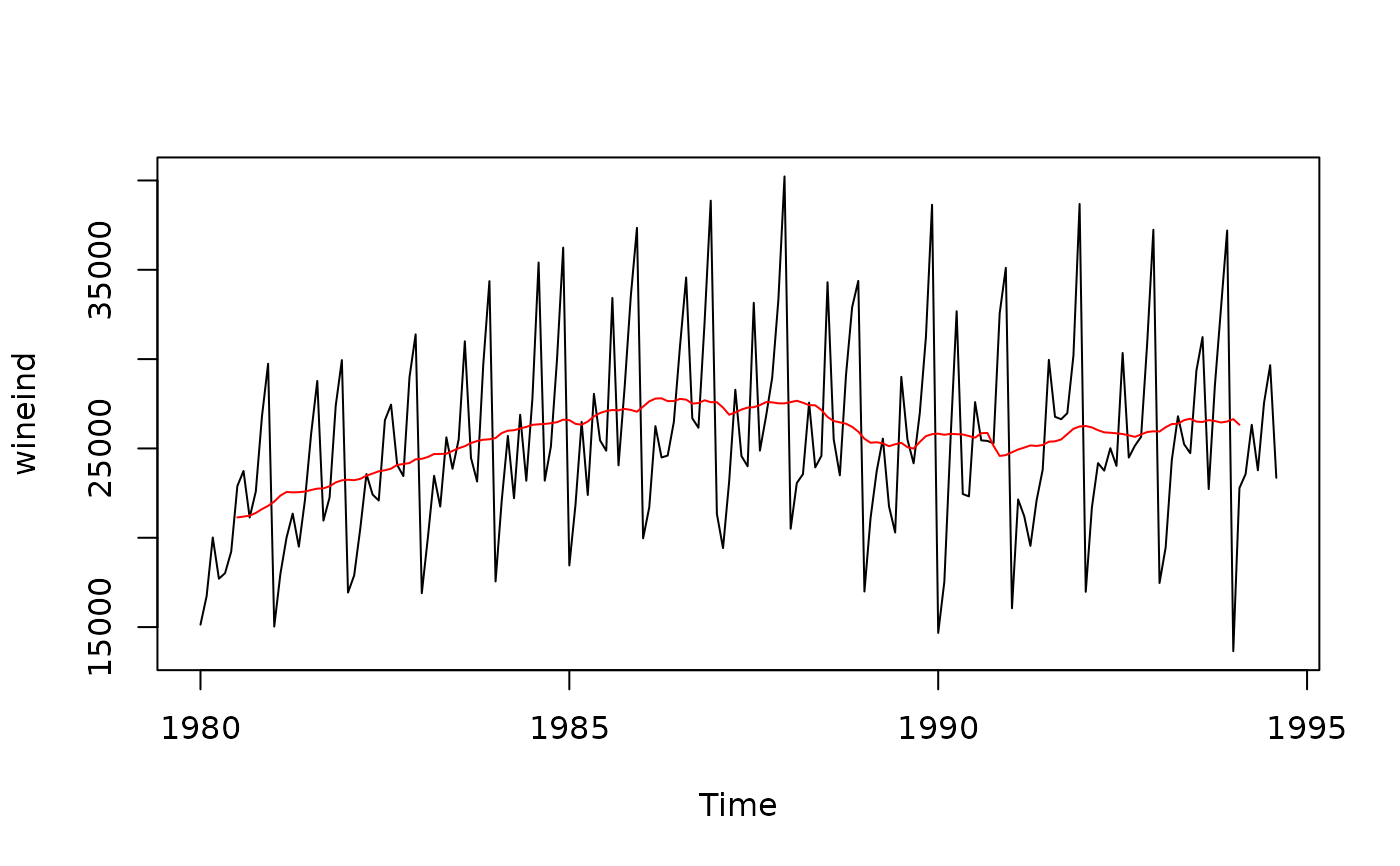ma computes a simple moving average smoother of a given time series.

## Usage

ma(x, order, centre = TRUE)

## Arguments

x

Univariate time series

order

Order of moving average smoother

centre

If TRUE, then the moving average is centred for even orders.

## Value

Numerical time series object containing the simple moving average smoothed values.

## Details

The moving average smoother averages the nearest order periods of each observation. As neighbouring observations of a time series are likely to be similar in value, averaging eliminates some of the randomness in the data, leaving a smooth trend-cycle component. $$\hat{T}_{t} = \frac{1}{m} \sum_{j=-k}^k y_{t+j}$$ where $$k=\frac{m-1}{2}$$

When an even order is specified, the observations averaged will include one more observation from the future than the past (k is rounded up). If centre is TRUE, the value from two moving averages (where k is rounded up and down respectively) are averaged, centering the moving average.

decompose

Rob J Hyndman

## Examples


plot(wineind)
sm <- ma(wineind,order=12)
lines(sm,col="red")